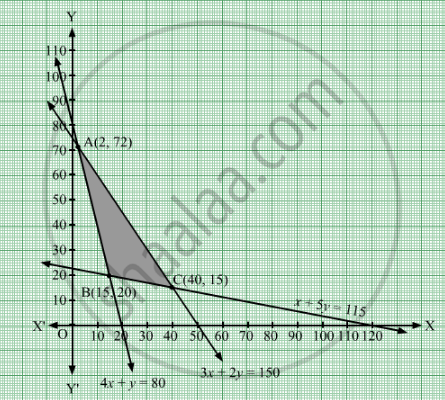Share
Notifications

View all notifications
Books Shortlist
Your shortlist is empty

# Solve the Following Linear Programming Problem Graphically: Minimize Z = 6 X + 3 Y Subject to the Constraints: 4 X + Y ≥ 80 X + 5 Y ≥ 115 3 X + 2 Y ≤ 150 X ≥ 0 , Y ≥ 0 - CBSE (Arts) Class 12 - Mathematics

Login
Create free account

Forgot password?
ConceptGraphical Method of Solving Linear Programming Problems

#### Question

Solve the following linear programming problem graphically:
Minimize  z = 6 x + 3 y
Subject to the constraints:

4 x + $y \geq$ 80
x + 5 $y \geq$ 115

3 x + 2 $y \leq$ 150
$x \geq$ 0  , $y \geq$ 0

#### Solution

The given constraints are

4x + y  $\geq$ 80

x + 5y  $\geq$  115

3x + 2y  $\geq$  150

x,y  $\geq$  0

Converting the given inequations into equations, we get
4x + y = 80, x + 5y = 115, 3x + 2= 150, x = 0 and = 0
These lines are drawn on the graph and the shaded region ABC represents the feasible region of the given LPP.It can be observed that the feasible region is bounded. The coordinates of the corner points of the feasible region are A(2, 72), B(15, 20) and C(40, 15).
The values of the objective function, Z at these corner points are given in the following table:

 Corner Point Value of the Objective Function Z = 6x + 3y A(2, 72) Z = 6 × 2 + 3 × 72 = 228 B(15, 20) Z = 6 × 15 + 3 × 20 = 150 C(40, 15) Z = 6 × 40 + 3 × 15 = 285

From the table, is minimum at = 15 and y = 20 and the minimum value of is 150.
Thus, the minimum value of Z is 150.

Is there an error in this question or solution?

#### Video TutorialsVIEW ALL 

Solution Solve the Following Linear Programming Problem Graphically: Minimize Z = 6 X + 3 Y Subject to the Constraints: 4 X + Y ≥ 80 X + 5 Y ≥ 115 3 X + 2 Y ≤ 150 X ≥ 0 , Y ≥ 0 Concept: Graphical Method of Solving Linear Programming Problems.
S